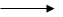Question 6

# How many mL of 0.1 M HCl are required to react completely with 1 g mixture of Na2CO3 and NaHCO3 containing equimolar amounts of both?

let the amount of Na2CO3 be x

& that of NaHCO3 be 1-x

Now moles of Na2CO3 = x / 106

& moles of NaHCO3 = 1-x / 84

Now according to question , number of moles of Na2Co3 = number of moles of NaHCO3

Therefore x / 106 = 1-x / 84

84x = 106-106x

84x +106x = 106

190x = 106

Or

x = 106 / 190 = 0.558

Therefore moles of Na2Co3 = 0.558 / 106 = 0.00526

&

moles of NaHCO3 = 1 - 0.558 / 84 = 0.0053

Now Hcl reacts with Na2Co3 & NaHCO3 as follows:

Na2Co3 + 2Hcl2Nacl + H2o + CO2

NaHCO3 + HclNacl + H2o + CO2

From the above reactions, 1 mol of Na2Co3 will react with 2 mol of Hcl

Therefore 0.00526 mol of Na2Co3 will react with 2 x 0.00526 mol of Hcl & similarly 0.00526 mol of NaHCO3 will react with 0.00526 mol of Hcl

Total moles of Hcl required to react with mixture of of NaHCO3 & Na2Co3

= 2 X 0.00526 + 0.00526 =0.01578 mol

Also according to question 0.1 mol of 0.1 M Hcl is present in 1000 ml

Or

0.01578 mol of 0.1 M Hcl is present in (1000/0.1) x 0.01578 = 157.8 ml

Hence, 158 mL of 0.1 M of HCl is required to react completely with 1 g mixture of Na2CO3 and NaHCO3, containing equimolar amounts of both.